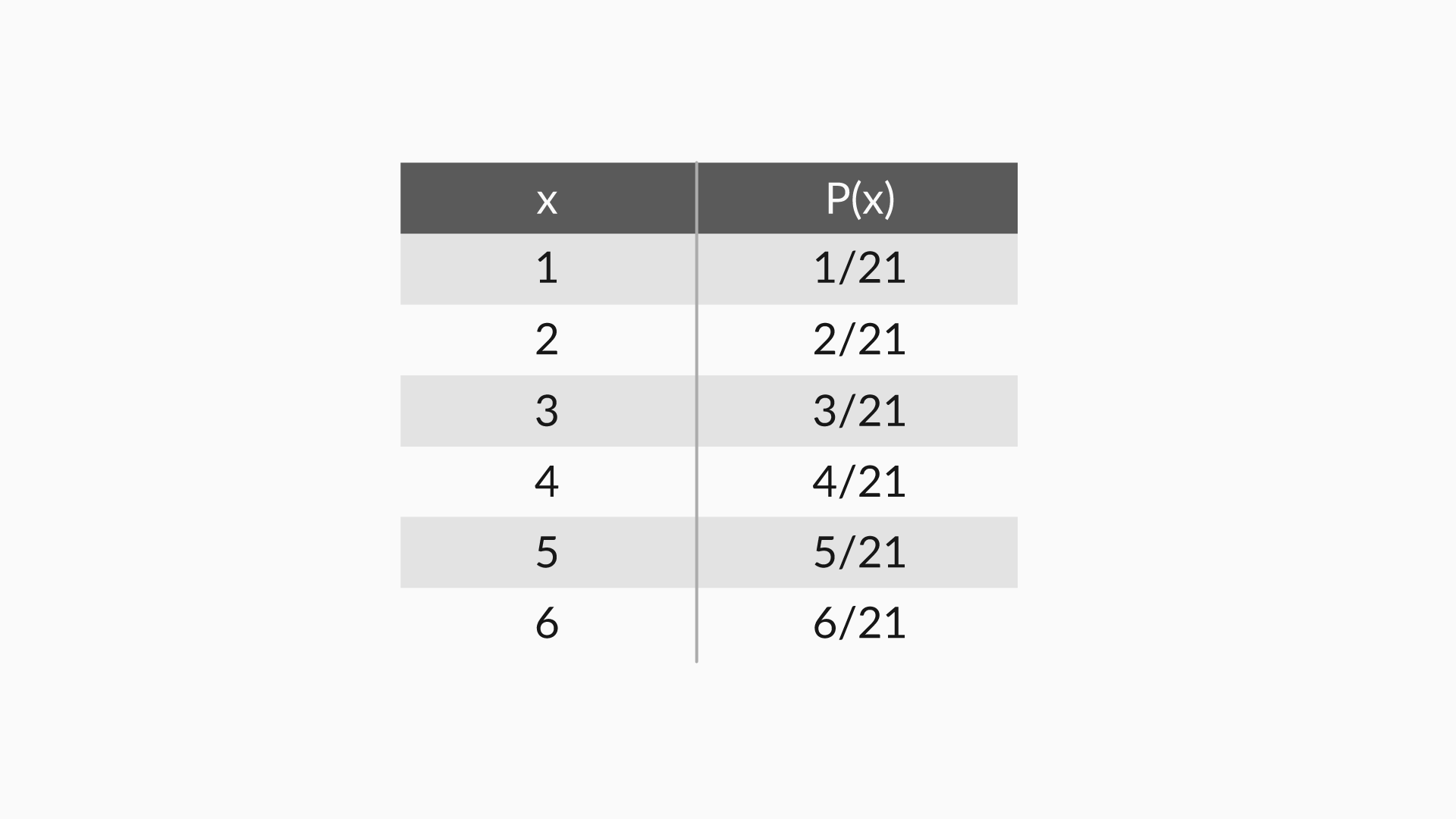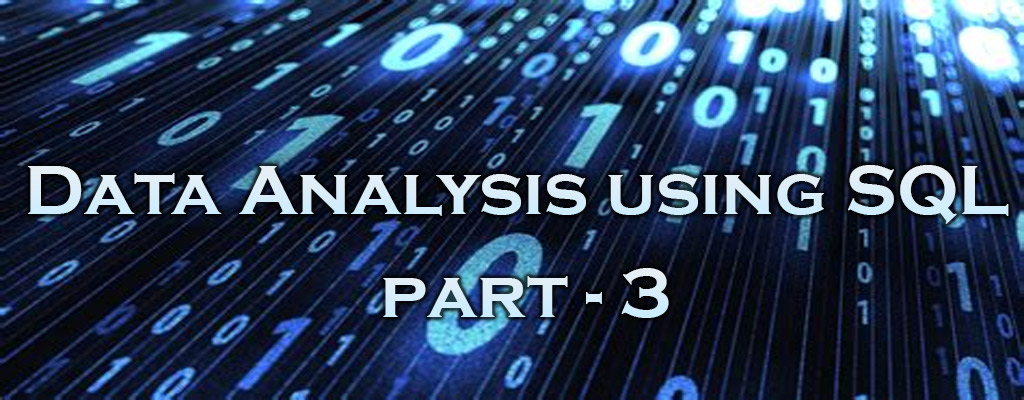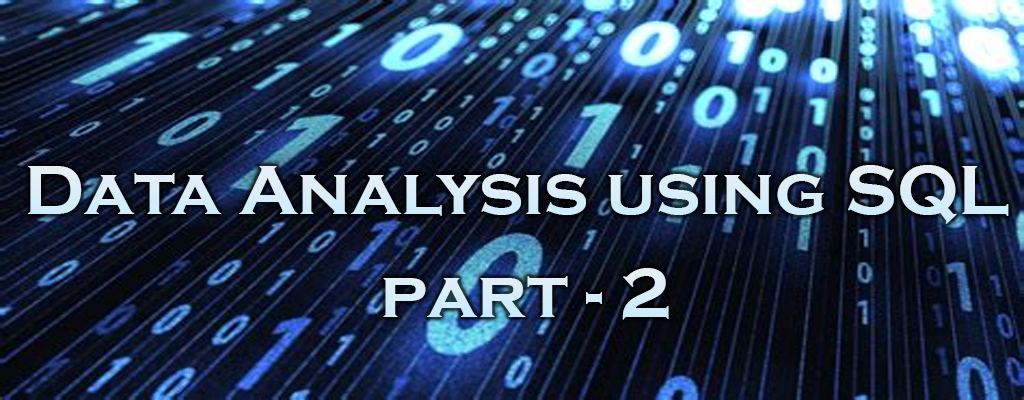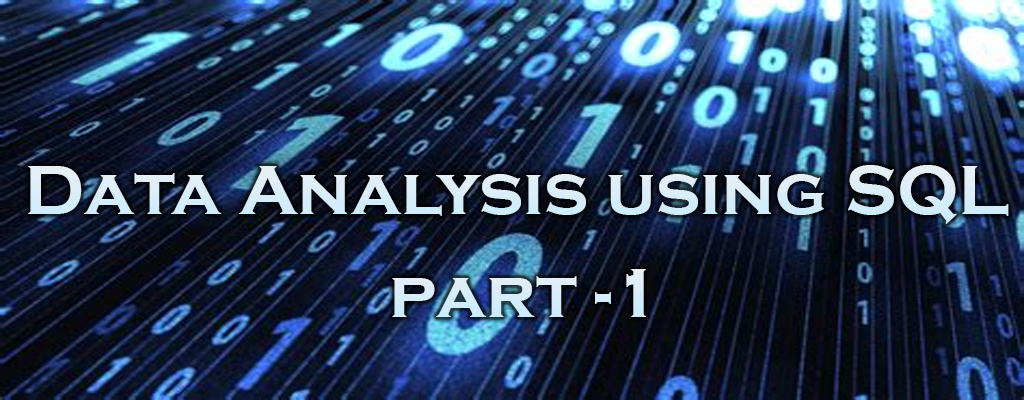# Linear Regression Interview Questions – Part 2

In the previous post, you saw some common interview questions asked on linear regression. The questions in that segment were mostly related to the essence of linear regression and focused on general concepts related to linear regression. This section extensively covers the common interview questions asked related to the concepts learnt in multiple linear regression.

Q1. What is Multicollinearity? How does it affect the linear regression? How can you deal with it?

Multicollinearity occurs when some of the independent variables are highly correlated (positively or negatively) with each other. This multicollinearity causes a problem as it is against the basic assumption of linear regression. The presence of multicollinearity does not affect the predictive capability of the model. So, if you just want predictions, the presence of multicollinearity does not affect your output. However, if you want to draw some insights from the model and apply them in, let’s say, some business model, it may cause problems.

# Linear Regression Interview Questions – Part 1

It is a common practice to test data science aspirants on linear regression as it is the first algorithm that almost everyone studies in Data Science/Machine Learning. Aspirants are expected to possess an in-depth knowledge of these algorithms. We consulted hiring managers and data scientists from various organisations to know about the typical Linear Regression questions which they ask in an interview. Based on their extensive feedback a set of question and answers were prepared to help students in their conversations.

Q1. What is linear regression?

In simple terms, linear regression is a method of finding the best straight line fitting to the given data, i.e. finding the best linear relationship between the independent and dependent variables.

# Logistic Regression Interview Questions – Part 3

Q1. What is accuracy?
Accuracy is the number of correct predictions out of all predictions made.

Accuracy=True Positives+True NegativesTotal Number of Predictions

Q2. Why is accuracy not a good measure for classification problems?
Accuracy is not a good measure for classification problems because it gives equal importance to both false positives and false negatives. However, this may not be the case in most business problems. For example, in the case of cancer prediction, declaring cancer as benign is more serious than wrongly informing the patient that he is suffering from cancer. Accuracy gives equal importance to both cases and cannot differentiate between them.

# Logistic Regression Interview Questions – Part 2

Q1. What is the Maximum Likelihood Estimator (MLE)?
The MLE chooses those sets of unknown parameters (estimator) that maximise the likelihood function. The method to find the MLE is to use calculus and setting the derivative of the logistic function with respect to an unknown parameter to zero, and solving it will give the MLE. For a binomial model, this will be easy, but for a logistic model, the calculations are complex. Computer programs are used for deriving MLE for logistic models.

(Here’s another approach to answering the question.)

# Logistic Regression Interview Questions – Part 1

Q1. What is a logistic function? What is the range of values of a logistic function?
The logistic function is as defined below:

f(z)=1(1+e−z)

The values of a logistic function will range from 0 to 1. The values of Z will vary from −∞ to +∞.

# Inferential Statistics – Part 1## Introduction: Inferential Statistics :

The process of “inferring” insights from sample data is called “Inferential Statistics”

### Basics of Probability:

In the topic we will go through:

• Basic definition of probability
• Multiplication rule of probability
• nCr (Combinatorics)

### Random Variables

Random variables are quantities with distinct characteristics and behavior.

1. Random variables are denoted by capital letters
2. Random variables are associated with random processes
3. Random variables give numbers to outcomes of random events.

# Data Analysis using SQL – Part 3 – Advanced SQLYou now know two types of SQL commands, namely:

• Data Definition Language
• Data Manipulation Language

The Data Definition Language (DDL) is used to create and modify the schema of the database. Commands like CREATE, ALTER and DROP are part of this language.

As a data analyst, you would always be actively involved in data retrieval activities. Here, the Data Manipulation Language (DML) commands would come in handy, e.g. the DML command SELECT, its purpose, various clauses and filtering operations.

### Order by Clause

The SQL ORDER BY clause is used to sort the data in ascending or descending order, based on one or more columns. Some databases sort the query results in an ascending order by default.

The basic syntax of the ORDER BY clause is as follows −

```SELECT column-list
FROM table_name
[WHERE condition]
[ORDER BY column1, column2, .. columnN] [ASC | DESC];
```

The order in which it appears is “select * from table where some_variable = x order by some_variable”.

# Data Analysis using SQL – Part 2 – Database design## Defining Data Warehouse

A data warehouse would be a central repository of the data of the entire enterprise.

A data warehouse is a collection of data. It exhibits the following properties:

• Subject-oriented: A data warehouse should contain information about a few well-defined subjects rather than containing information about the entire enterprise.
• Integrated: A data warehouse is an integrated repository of data. It contains information from various systems within an organisation.
• Non-volatile: Data values cannot be changed without a valid reason.
• Time-variant: A data warehouse contains historical data for analysis.

## Structure of Data Warehouse

One of the primary methods of designing a data warehouse is called dimensional modelling.

The two key elements of dimensional modelling are facts and dimensions, which are basically different types of variables used to design a warehouse. They are arranged together in a specific way known as a schema diagram.

## OLAP vs. OLTP

### What is OLAP?

Online Analytical Processing, a category of software tools which provide analysis of data for business decisions. OLAP systems allow users to analyze database information from multiple database systems at one time.

The primary objective is data analysis and not data processing.

### What is OLTP?

Online transaction processing shortly known as OLTP supports transaction-oriented applications in a 3-tier architecture. OLTP administers day to day transaction of an organization.

The primary objective is data processing and not data analysis

### Example of OLAP

Any Datawarehouse system is an OLAP system. Uses of OLAP are as follows

• A company might compare their mobile phone sales in September with sales in October, then compare those results with another location which may be stored in a sperate database.
• Amazon analyzes purchases by its customers to come up with a personalized homepage with products which likely interest to their customer.

### Example of OLTP system

An example of OLTP system is ATM center. Assume that a couple has a joint account with a bank. One day both simultaneously reach different ATM centers at precisely the same time and want to withdraw total amount present in their bank account.

However, the person that completes authentication process first will be able to get money. In this case, OLTP system makes sure that withdrawn amount will be never more than the amount present in the bank. The key to note here is that OLTP systems are optimized for transactional superiority instead data analysis.

Other examples of OLTP system are:

• Online banking
• Online airline ticket booking
• Sending a text message
• Order entry
• Add a book to shopping cart

## Star Schema

Facts and dimensions are the two key elements of dimension modelling. A typical problem might involve multiple databases with many different variables and we may not be interested in all the variables. Hence, only some facts and dimensions are combined in a specific manner to create the structure of data warehouse. This structure is called as a schema diagram

A schema is an outline of the entire data warehouse. It shows how different data sets are connected and the different attributes of each data being used for the data warehouse.

# Summary

You learnt about what a data warehouse is and the difference between a data warehouse and a transactional database. You learnt that OLAP systems are Subject-oriented, Integrated, Non-volatile and Time variant.

You learnt that the data warehouse gives an integrated view of the entire organisation and the data is organised for efficiently carrying out analysis. You also learnt the difference between a data warehouse and a transactional database.

You also learnt about facts and dimensions. You also learnt how to arrange facts and dimensions to design a data warehouse. You saw how dimension tables act as the metadata that is the data about data and they enhance the facts table to enhance insights about the data.

# Data Analysis using SQL – Basics of SQL – Part 1### An introduction to RDBMS and SQL

There are various ways to arrange and manage data in a database. The most common is to arrange the data in tables, which is similar to an Excel file. The table contains multiple columns and rows.

A Database is a collection of related data. But a question still remains unanswered — how do you access this data? The answer is a specific language designed for this purpose, called the Structured Query Language, or SQL.

## Concepts

Tables − In relational data model, relations are saved in the format of Tables. This format stores the relation among entities. A table has rows and columns, where rows represents records and columns represent the attributes.

Tuple − A single row of a table, which contains a single record for that relation is called a tuple.

Relation instance − A finite set of tuples in the relational database system represents relation instance. Relation instances do not have duplicate tuples.